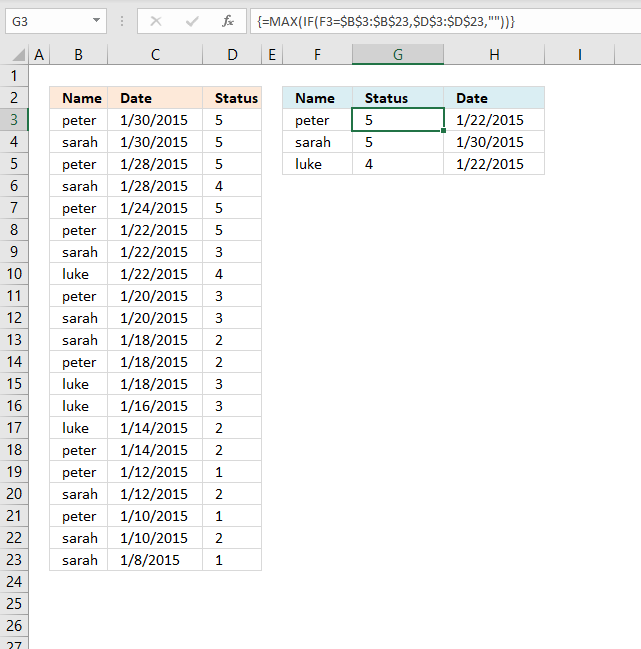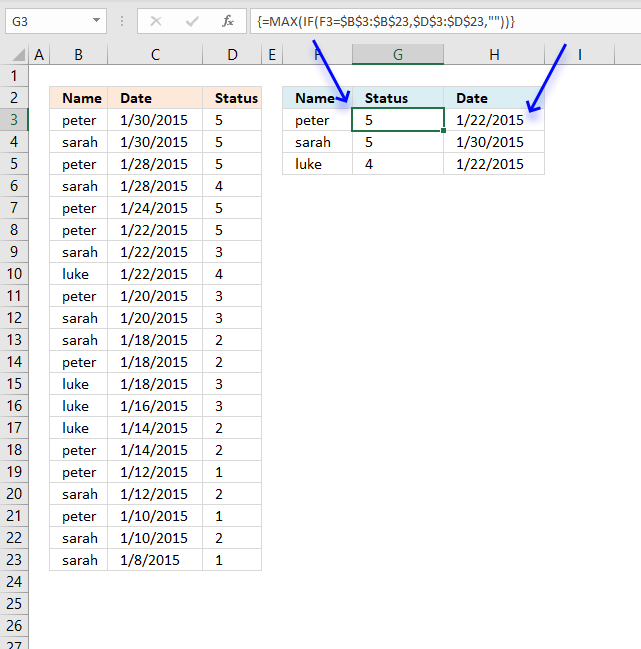Author: Oscar Cronquist Article last updated on May 21, 2021This article describes how to filter records based on the maximum value of a specific item. There are names in column B, dates in column C, and numbers in column D.

The formula in cell G3 extracts numbers from column D based on the given condition in cell F3. The highest number is then calculated and returned to cell G3 with the corresponding date in column H.

Hello Oscar,
thank you for sharing you knowledge and helping us with these excellent formulas.
I have a case i could really need your help with:
The following Table shows a history of names, stati and the date the status was acquired. BUT: people can acquire the same status more than just once (or at least report it). Now I want to know, when each person (peter, sarah & luke) have acquired their individual highest status.Name Date Status
peter 30.01.2015 5
sarah 30.01.2015 5
peter 28.01.2015 5
sarah 28.01.2015 4
peter 24.01.2015 5
peter 22.01.2015 5
sarah 22.01.2015 3
luke 22.01.2015 4
peter 20.01.2015 3
sarah 20.01.2015 3
sarah 18.01.2015 2
peter 18.01.2015 2
luke 18.01.2015 3
luke 16.01.2015 3
luke 14.01.2015 2
peter 14.01.2015 2
peter 12.01.2015 1
sarah 12.01.2015 2
peter 10.01.2015 1
sarah 10.01.2015 2
sarah 08.01.2015 1answers have to be :
Names latest status in status since
Peter 5 22.01.2015
sarah 5 30.01.2015
luke 4 22.01.2015I would really appreciate your help.
Greetings.Array formula in G3:

=MAX(IF(F3=\$B\$3:\$B\$23, \$D\$3:\$D\$23, ""))

Array formula in cell H3:

=MIN(IF((MAX(IF(F3=\$B\$3:\$B\$23, \$D\$3:\$D\$23, ""))=\$D\$3:\$D\$23)*(F3=\$B\$3:\$B\$23),\$C\$3:\$C\$23, "A"))

### How to enter an array formula

1. Select cell F2.
2. Paste formula in formula bar.
3. Press and hold CTRL + SHIFT simultaneously.
4. Press Enter once.
5. Release all keys.

This is what the formula in the formula bar looks like:

{=MAX(IF(E2=\$A\$2:\$A\$22, \$C\$2:\$C\$22, ""))}

Don't enter the curly brackets yourself, they appear automatically when you press CTRL + SHIFT + ENTER, see steps above.

Update 2021-05-20, The MAXIFS and MINIFS functions were introduced in Excel 2016. Enter this formula as regular formula in cell G3:

=MAXIFS(D3:D23, B3:B23, F3)

Excel 2016 formula in cell H3:

=MINIFS(C3:C23, D3:D23, G3,B3:B23, F3)

These two formulas are regular formulas.

### Explaining array formula in cell G3

#### Step 1 - Find name in cell E2 in cell range B2:B22

The equal sign lets you compare a value to another value, you can also compare a value to an entire array of values. This is what we are going to do here.

The equal sign is one of many logical operators and the result is a boolean value TRUE or FALSE, or their numerical equivalents 1 (TRUE) or 0 (FALSE).

F3=\$B\$3:\$B\$23

becomes

"peter"={"peter"; "sarah"; "peter"; "sarah"; "peter"; "peter"; "sarah"; "luke"; "peter"; "sarah"; "sarah"; "peter"; "luke"; "luke"; "luke"; "peter"; "peter"; "sarah"; "peter"; "sarah"; "sarah"}

and returns

{TRUE; FALSE; TRUE; FALSE; TRUE; TRUE; FALSE; FALSE; TRUE; FALSE; FALSE; TRUE; FALSE; FALSE; FALSE; TRUE; TRUE; FALSE; TRUE; FALSE; FALSE}

#### Step 2 - Replace TRUE with corresponding value in cell range C2:C22 and FALSE with nothing

The IF function returns one value if the logical test is TRUE and another value if FALSE.

IF(logical_test, [value_if_true], [value_if_false])

IF(F3=\$B\$3:\$B\$23, \$D\$3:\$D\$23, "")

becomes

IF({TRUE; FALSE; TRUE; FALSE; TRUE; TRUE; FALSE; FALSE; TRUE; FALSE; FALSE; TRUE; FALSE; FALSE; FALSE; TRUE; TRUE; FALSE; TRUE; FALSE; FALSE}, \$C\$2:\$C\$22, "")

becomes

IF({TRUE; FALSE; TRUE; FALSE; TRUE; TRUE; FALSE; FALSE; TRUE; FALSE; FALSE; TRUE; FALSE; FALSE; FALSE; TRUE; TRUE; FALSE; TRUE; FALSE; FALSE}, {5; 5; 5; 4; 5; 5; 3; 4; 3; 3; 2; 2; 3; 3; 2; 2; 1; 2; 1; 2; 1}, "")

and returns

{5; ""; 5; ""; 5; 5; ""; ""; 3; ""; ""; 2; ""; ""; ""; 2; 1; ""; 1; ""; ""}

#### Step 3 - Find largest value in array

The MAX function returns the largest number froma cell range or an array.

MAX(IF(F3=\$B\$3:\$B\$23, \$D\$3:\$D\$23,""))

becomes

MAX({5; ""; 5; ""; 5; 5; ""; ""; 3; ""; ""; 2; ""; ""; ""; 2; 1; ""; 1; ""; ""})

and returns 5 in cell F2.

### Explaining array formula in cell H3#### Step 1 - Compare largest value with cell range \$D\$3:\$D\$23

This step returns an array containing boolean values, TRUE if they meet the condition and FALSE if not.

MAX(IF(F3=\$B\$3:\$B\$23, \$D\$3:\$D\$23, ""))=\$D\$3:\$D\$23

becomes

5=\$D\$3:\$D\$23

and returns

{TRUE; TRUE; TRUE; FALSE; TRUE; TRUE; FALSE; FALSE; FALSE; FALSE; FALSE; FALSE; FALSE; FALSE; FALSE; FALSE; FALSE; FALSE; FALSE; FALSE; FALSE}

#### Step 2 - Compare value in cell F3 with cell range \$B\$3:\$B\$23

This step compares the name in cell F3 to the values in cell range \$B\$3:\$B\$23.

F3=\$B\$3:\$B\$23

returns

{TRUE; FALSE; TRUE; FALSE; TRUE; TRUE; FALSE; FALSE; TRUE; FALSE; FALSE; TRUE; FALSE; FALSE; FALSE; TRUE; TRUE; FALSE; TRUE; FALSE; FALSE}

#### Step 3 - Multiply array in step 1 with array in step 2

This step applies AND logic meaning both conditions must be met in order to return TRUE.

TRUE * TRUE = TRUE (1)

TRUE * FALSE = FALSE (0)

FALSE * TRUE = FALSE (0)

FALSE * FALSE = FALSE (0)

When you multiply boolean values you always get the numerical equivalent, TRUE = 1 and FALSE = 0 (zero)

MIN(IF((MAX(IF(F3=\$B\$3:\$B\$23, \$D\$3:\$D\$23, ""))=\$D\$3:\$D\$23)*(F3=\$B\$3:\$B\$23),\$C\$3:\$C\$23, "A"))

MAX(IF(E2=\$A\$2:\$A\$22,\$C\$2:\$C\$22,""))=\$C\$2:\$C\$22)*(E2=\$A\$2:\$A\$22)

becomes

{TRUE; TRUE; TRUE; FALSE; TRUE; TRUE; FALSE; FALSE; FALSE; FALSE; FALSE; FALSE; FALSE; FALSE; FALSE; FALSE; FALSE; FALSE; FALSE; FALSE; FALSE} * {TRUE; FALSE; TRUE; FALSE; TRUE; TRUE; FALSE; FALSE; TRUE; FALSE; FALSE; TRUE; FALSE; FALSE; FALSE; TRUE; TRUE; FALSE; TRUE; FALSE; FALSE}

and returns

{1; 0; 1; 0; 1; 1; 0; 0; 0; 0; 0; 0; 0; 0; 0; 0; 0; 0; 0; 0; 0}

#### Step 4 - Replace TRUE with corresponding value in cell range C2:C22 and FALSE with random text string

The IF function returns one value if the logical test is TRUE and another value if FALSE.

IF(logical_test, [value_if_true], [value_if_false])

IF(E2=\$A\$2:\$A\$22,\$C\$2:\$C\$22,""))=\$C\$2:\$C\$22)*(E2=\$A\$2:\$A\$22),\$B\$2:\$B\$22,"A")

bcomes

IF({1; 0; 1; 0; 1; 1; 0; 0; 0; 0; 0; 0; 0; 0; 0; 0; 0; 0; 0; 0; 0},\$B\$2:\$B\$22,"A")

and returns

{42034; "A"; 42032; "A"; 42028; 42026; "A"; "A"; "A"; "A"; "A"; "A"; "A"; "A"; "A"; "A"; "A"; "A"; "A"; "A"; "A"}

#### Step 5 - Find smallest value in array, text strings are ignored

The MIN function returns the smallest number in a cell range or array, text strings are ignored.

MIN(IF((MAX(IF(E2=\$A\$2:\$A\$22,\$C\$2:\$C\$22,""))=\$C\$2:\$C\$22)*(E2=\$A\$2:\$A\$22),\$B\$2:\$B\$22,"A"))

becomes

MIN({42034; "A"; 42032; "A"; 42028; 42026; "A"; "A"; "A"; "A"; "A"; "A"; "A"; "A"; "A"; "A"; "A"; "A"; "A"; "A"; "A"})

and returns 42026 in cell G2.

### Get the Excel fileDenis-1.xlsx